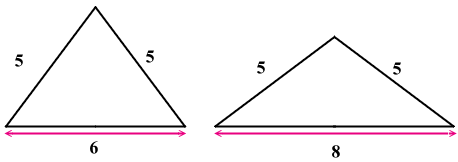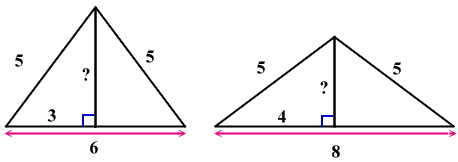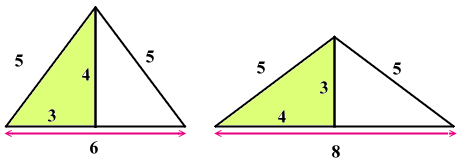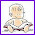### A triangle problem to solve

I have another neat problem for you to solve. This one should be accessible to middle schoolers on up.

The sides of triangles A and B measure 5, 5, 8 and 5, 5, 6 respectively. What is the ratio of the area of triangle A to that of triangle B? Express in simplest a:b form.

This problem is original to John Morse. He is a local mathematics researcher/
author/tutor/computer programmer in Delmar, NY, and has written this problem to help learners use creative and problem-solving skills in various ways. And I think this problem can indeed help in that - it can be solved in many different ways.

[update - solution follows]

I like this problem because there are many ways to solve it and to use it with different grade-level students - such as is already mentioned in the comments.

1) You could use this as a drawing and measuring exercise with 6th or 7th graders who have learned to do compass and ruler constructions.

Once they know how to construct a triangle given its three sides (or see here as well), they can construct these two, then draw & measure the altitudes, and calculate the areas.

Of course the downside is that measurements are bound to be inaccurate, and so they are unlikely to get exactly 12 square units as the area. But then again, you could discuss this topic as well (inaccuracy of measurements).

2) Another way to (clumsily) solve this is to calculate the areas of the two triangles using Heron's formula (if you know it exists!) Just plug the numbers in.

3) The elegant way... accessible to students who know about Pythagorean Theorem. I'll take you through it step by step.

The first step is to NOTICE that the triangles are isosceles ("same-legged"), and to draw a sketch.

(Mine is not a "rough sketch" but as exact as can be done in PhotoImpact, because I started out by drawing the three sides with the given lengths. Then I calculated one angle, and turned one side in that angle to get a "corner".)

In order for the students to easily see the solution, it is fairly important to be able to sketch the two triangles.

When drawing isosceles triangles, think of the capital letter "A" and its two "legs". We just need to draw one that "stands" taller and one that "stands" with its legs wider open.

Notice one of them is close to an equilateral triangle since it's sides are "almost" the same.. 5, 5, and 6. So you would sketch it as almost an equilateral triangle, just make those "legs" to open a little more. Then the 5-5-8 triangle needs to open its "legs" quite wide to make for the wide 8-unit base.Now, we are concerned about their AREAS, so naturally we need an altitude. Once you draw the two altitudes, the idea is usually to find how many units long the altitudes are, so you could calculate the area.

But in this case, there's sort of a shortcut. You will realize (or remember) that the altitude forms two little RIGHT triangles in each triangle.In the triangle on the left, the two known side lengths of the little triangle are 5 and 3, and in the other the two known lengths are 5 and 4.

This would HOPEFULLY ring a bell.... DING! REMEMBER the 3-4-5 right triangle? Would it fit here?

Oh yes, it does. In BOTH triangles, the little triangles are 3-4-5 right triangles!

To prove it, you CAN use the Pythagorean theorem: in one little triangle, you'd have x, 4, and 5 (5 being the hypotenuse), so solving x2 + 42 = 52 gives you x = 3, and in the other triangle, solving x2 + 32 = 52 gives you x = 4.

But there's no need to go through the solving if you remember that 3-4-5 is a right triangle.OK, now we know the altitudes and could calculate the areas... BUT wait! If the little triangles are identical (congruent), then their areas are the same, and the areas of the large triangles are the same as well. We only need to find the ratio of the two areas, not the actual areas.

So with two triangles with the same area, the ratio is of course 1:1.mathmom said…
I like that problem! When is the Pythagorean theorem usually taught? I think of that as being Algebra I. (My way uses it, or at least uses special triangles related to it.)Unknown said…
The areas will be equal i.e. 1:1For more difficult triangle pairs like 5,6,7 and 5,6,8 you'd want to use either the Law of Cosines, or Heron's formula, which can be applied to this problem, but are more cumbersome than necessary for two isosceles triangles.

BTW, if your pupil can prove the Law of Cosines, using unit-circle trig, it's a beautiful doorway to the cosine and sine addition and subtraction relationships.SplineGuy said…
Good question. I might offer some followup questions to promote deeper discussions among my students.

Are there only two heights for which isosceles triangles of these type have the same area?

Is it always two? Why or why not?

This can tie quadratic equations to geometry and trigonometry, helping students to see connections.This type of problem shows that the two different parent isosceles triangles can be broken down into (or constructed from) congruent right triangles.

Other examples include 13, 13 10 and 13, 13, 24;
17 17 30 and 17 17 16; 25 25 14 and 25 25 48
as well as their multiples,

as well as triangles that involve irrational numbers such as equilateral a-a-a and isosceles
a-a-a(sq rt 3)Unknown said…
i love this type of problems. and the answer is = 2*square root2:1 by using herons formula.Maria Miller said…
Abhi,
I assume you've made a mistake somewhere... it's already shown the ratio is 1:1, or in other words the areas are equal.Educosoft News said…
I like the problem. If you want the solution of different mathematics problems and wants to learn mathematics in an interactive manner, Just visit the website
www.educosoftregister.com.
View the Demo files available their, and register yourself to use the system.